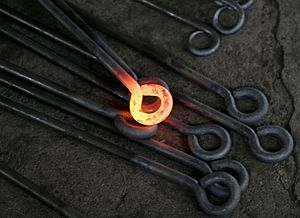# Heat facts for kids

Kids Encyclopedia Facts
(Redirected from Hot)

Heat is the opposite of cold. In thermodynamics, heat means energy which is moved between two things when one of them is hotter than the other.

Heat is not the same as temperature. The temperature of an object is a measure of the average speed of the moving particles it is made from and the energy of the particles is called the internal energy. When an object is heated, its internal energy can increase to make the object hotter. The first law of thermodynamics says that the increase in internal energy is equal to the heat added minus the work done on the surroundings.

Thermal energy is the type of energy that a thing has because of its temperature. In thermodynamics, thermal energy is the internal energy present in a system in a state of thermodynamic equilibrium because of its temperature. That is, heat is defined as a spontaneous flow of energy (energy in transit) from one object to another, caused by a difference in temperature between two objects; so objects do not possess heat.

## Properties of HeatA red-hot iron rod from which heat transfer to the surrounding environment will be primarily through radiation.

Heat is a form of energy and not a physical substance. Heat has no mass.

Heat can move from one place to another in different ways:

The measure of how much heat is needed to cause some change in temperature for a material is the specific heat capacity of the material. If the particles in the material are hard to move, then more energy is needed to make them move quickly, so a lot of heat will cause a small change in temperature. A different particle that is easier to move will need less heat for the same change in temperature.

Unless some work is done, heat moves only from hot things to cold things.

## Measuring Heat

Heat can be measured. That is, the amount of heat given out or taken in can be given a value. One of the units of measurement for heat is the joule.

Heat is usually measured with a calorimeter, where the energy in a material is allowed to flow into nearby water, which has a known specific heat capacity. The temperature of the water is then measured before and after, and heat can be found using a formula.

## Images for kidsHeat Facts for Kids. Kiddle Encyclopedia.